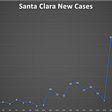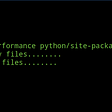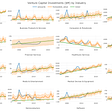# Basics of Machine Learning — Linear Regression (The very first topic of your Data Science Career)

Linear Regression has 2 parts:

• Simple Linear Regression
• Multiple Linear Regression

Simple means 1 input & 1 output, Linear means Straight/Direct and Regression means a measure of the relation between the one variable (output) and corresponding values of other variables (input).

SLR is expressed as: y = mx + b

where:

y = output

x = input

m = slope

b = constant

Simple Linear Regression:

To put it in the most simplest way, we have 1 input variable and 1 output variable. Both these variables are associated with each other by a single line having a linear relationship or we can say they have direct relationship with each other. Now this relationship can be either positive or negative in nature.

We will always express this relationship as the Best Fit Line:

The best fit line is a straight line that is the best approximation of the given set of data. The equation for the best fitting line is,

The above image shows the Linear and Non Linear Relationship. The red line is the Best Fit Line.

The line that fits the data best will be the one for which the n prediction errors (one for each observed data point) are as small as possible in some overall sense.

We use various metrics to determine the goodness of fit:

• R2(R-squared) or Coefficient of Determination
• Root Mean Squared Error
• Residual Standard Error

R2(R-squared) or Coefficient of Determination:

Mathematically:

a. Residual Sum of Squares (RSS) is the measure of the difference between the expected and the actual output. A small RSS indicates a tight fit of the model to the data. Mathematically RSS is,

b. Total Sum of Squares (TSS) is defined as the sum of errors of the data points from the mean of the response variable. Mathematically TSS is,

The following figure shows the significance of R2:

Root Mean Squared Error:

The Root Mean Squared Error is the square root of the variance of the residuals. It indicates the absolute fit of the model to the data i.e. how close the observed data points are to the model’s predicted values. Mathematically it can be represented as

Residual Standard Error

To eliminate the biasness from the above estimate, we will divide the sum of squared residual by the degree of freedom rather than the total number of datapoints in the model. This term is then called the Residual Standard Error. Mathematically it can be represented as,

We will discuss the a very important topic ie. Assumption of Simple Linear Regression in the next Article.

I would love to get your feedback on this article or send me any queries you have on shobhit.bhargava3@gmail.com

Thanks and see you soon!!! Stay safe :)

--

--

--

## More from Shobhit Bhargava

Entrepreneurial/Market Research/Data Scientist/Tableau/SQL

Love podcasts or audiobooks? Learn on the go with our new app.

## Consider the Source: Climate Metrics and Their Underlying Models## Is the curve flattening here in the Bay Area?## Learn how to use pandas inplace parameter once and for all## 3 Simple Outlier/Anomaly Detection Algorithms every Data Scientist needs## ‘Conspirituality’ — the overlap between the New Age and conspiracy beliefs## Analysis/Prediction Model for the Production Awards Program for Documentary Filmmaking## Decoding the performance secret of world’s most popular Data Science library— Numpy## Exploratory v3.3 Released!## Shobhit Bhargava

Entrepreneurial/Market Research/Data Scientist/Tableau/SQL

## A Medic’s Machine Learning Diary: Day 6## So…I’m gonna become a data scientist. In a month😱## 8 Principles to Become an Influential Data Scientist